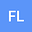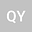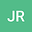Extremal values of vertex--degree--based topological indices over fluoranthene-type benzenoid systems with equal number of edges
•••Qingfang Ye
Ningbo University of Finance & Economics
Author ProfileLet $G$ be a graph with $n$ vertices. A vertex–degree–based topological index is defined from a set of real numbers $\{\psi_{ij}\}$ as $$TI(G)=\sum_{1\leq i\leq j\leq n-1}m_{ij}\psi_{ij},$$ where $m_{ij}$ is the number of edges of $G$ connecting a vertex of degree $i$ with a vertex of degree $j$. Many of the well–known topological indices are particular cases of this expression, including all Randi\’{c}-type connectivity indices. In this work we determine extremal values for $TI$ over the set of fluoranthene–type benzenoid systems with a fixed number of edges. The main idea consists in constructing fluoranthene–type benzenoid systems with maximal number of inlets in $\Gamma_{m}$ which have simultaneously minimal number of hexagons, where $\Gamma_{m}$ is the set of fluoranthene–type benzenoid systems with exactly $m(m\geq19)$ edges.